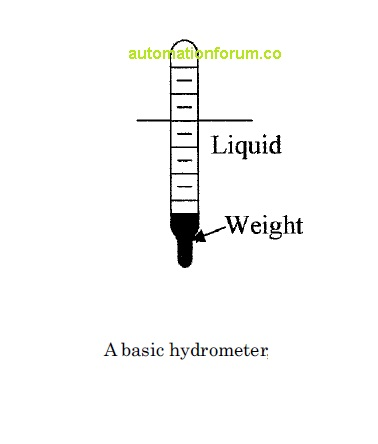# Density measuring devices## Introduction

In this session we are going to discuss about density measuring devices ,before that we need to go through the basics.

• Density ρ  of a material is defined as the mass per unit volume. Units of density are pounds (slug) per cubic foot [lb (slug)/ft3] or kilogram per cubic meter (kg/m3).
• Specific weight γ is defined as the weight per unit volume of a material, i.e., pounds per cubic foot (lb/ft3) or newton per cubic meter (N/m3). Specific gravity (SG) of a liquid or solid is defined as the density of the material divided by the density of water or the specific weight of the material divided by the specific weight of water at a specified temperature
• The relation between density and specific weight is given by γ=ρg

### Hydrometers

Hydrometers are the simplest device for measuring the specific weight or density of a liquid. The device consists of a graduated glass tube, with a weight at one end, which causes the device to float in an upright position. The device
sinks in a liquid until an equilibrium point between its weight and buoyancy is reached. The specific weight or density can then be read directly from the graduations on the tube. Such a device is shown in Figure

###Induction hydrometers

Induction hydrometers are used to convert the specific weight or density of a liquid into an electrical signal. In this case, a fixed volume of liquid set by the overflow tube is used in the type of setup shown in Figure, the displacement device, or hydrometer, has a soft iron or similar metal core attached. The core is positioned in a coil which forms part of a bridge circuit. As the density/specific weight of the liquid changes, the buoyant force on the displacement device changes. This movement can be measured by the coil and converted into a density reading.Vibration sensors

Vibration sensors are an alternate method of measuring the density of a fluid. Fluid is passed through a U tube which has a flexible mount so that it can vibrate when driven from an outside source. The amplitude of the vibration decreases as the specific weight or density of the fluid increases, so that by measuring the vibration amplitude the specific weight/density can be calculated.

###Differential bubblersDifferential bubblers can be used to measure liquid density or specific weight. Figure  shows the setup using a bubbler system. Two air supplies are used to supply two tubes whose ends are at different depths in a liquid, the difference in air pressures between the two air supplies is directly related to the density of the liquid by the following equation:where Δp is the difference in the pressures and Δh the difference in the height of the bottoms of the two tubes